### Quick description

When one is trying to find a proof of a mathematical statement, it can be surprisingly helpful to think about the converse of that statement as well. The reason is that an understanding of the converse can give important information about what a proof of the original statement would have to be like, thereby speeding up the search for it.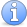Incomplete This article is incomplete. This article needs several more examples and some general discussion.

### Example 1

This may seem a slightly artificial example, but it came up recently in a research problem, and thinking about the converse was an essential step in finding a solution.

Suppose that you have a norm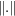on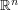and you would like to prove that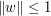for everybelonging to some subset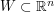. Suppose also that you want to do this by estimating the norms of at most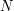points. (This situation occurred because the norm in question was randomly defined, and it was not possible to ask for too many events to occur simultaneously – at least if one wanted to avoid understanding the very subtle dependencies between those events.) The obvious method is to choose some subset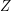of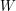consisting of at mostpoints, and to run an argument with the following general structure:

• every element ofcan be approximated (in a suitable sense) by an element of;

• if two elements ofare close (in that same sense) then their norms are close;

• the norm of every point inis smaller than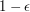.

Now let us think about whether this scheme of proof is necessary. That is, ifhas the property that if every point inhas a small norm then so does every point in, does it follow that every point incan be approximated, in some suitable sense, by a point in?

The answer is an emphatic no: one soon realizes that if the norm of every point inis at most 1, say, then the norm of every point in the convex hull ofis also at most 1. Armed with that observation, we can go back to the original problem with a potentially much more flexible method of proof:

• every element ofbelongs to the convex hull of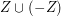;

• every element ofhas norm at most 1.

However, if we are sensible, we should learn our lesson and again investigate the converse. Suppose thatdoes not lie inside the convex hull of. Is it still possible that the norm restricted tocould control the norm restricted to?

The answer turns out to be no. If some point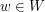lies outside the convex hull of, then the Hahn-Banach separation theorem implies that there is a linear functional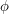such that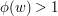, but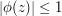for every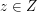. Thus, the seminorm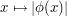is at most 1 everywhere onbut greater than 1 somewhere on. And providedis bounded we can easily convert that into a norm with the same property.

What we learn from this is that if we want to find a setand deduce from the fact that the norm of every vector inis small that the norm of every vector inis small, then, unless we know, and can use, further information about the norm(which in our problem we could not), we are forced to use the second method above. Thus, we can stop wasting time searching for alternative approaches.